Before continuing I recommend checking these out – basics on plotting using Python:

http://www.ucs.cam.ac.uk/docs/course-notes/unix-courses/pythontopics/graphs.pdf

http://www.ast.uct.ac.za/~sarblyth/pythonGuide/PythonPlottingBeginnersGuide.pdf

http://www.randalolson.com/2014/06/28/how-to-make-beautiful-data-visualizations-in-python-with-matplotlib/

NOTE: all of the above links save the plots to the screen, so you need some sort of Xserver or Desktop system for that. But sometimes your data collector & plotter is nothing more than a server without a monitor. So instead of plotting to a screen we need to plot to a file and then send that file over to a webserver for later viewing.

There are 3 steps in collecting and graphing data. I can generalize them as this:

Step 1. Generate / Collect Data into a file (via bash)
– The general format is that each line has the data from 1 point in time. Or if time is not represented, then each line represents whatever the x axis is (usually with data collection that is date/time)
Step 2. Repeat 1 as many times as needed (via cron)

Step 3. Graph data (can be done after 2 or after 1 or at anytime after *)
a. Read Collected Data (via python)
b. Parse Collected Data into variables (x and y for the graph) (via python)
c. Graph the variables to a plot/graph (via python)
d. Save the plot/graph to a file (via python)
e. Send plots to webserver (optional) (via bash or python)

* Graphing of data just needs to happen as long as there is 2 or more data points.

In this example we will go through each step using an example. Then at the end there will be extra examples of step 3 of graphing different types of collected data – which is the more complex step.

## *** FIRST EXAMPLE ***

#### 0. Get all of the tools needed for the job

Step 0 was not included in the generalization at the top as this is only required once. Here we will download the needed programs to get this done.

COMMANDS:

For later use in the python script (which will do the plotting), we need to get the name of default font – or else everytime the python script is ran a warning is shown that some font wasnt found so the system is using the some default go-to font. This warning can be avoided by setting the general font of the plotter to the default go-to font of the system.

#### 1. Generate / Collect Data:

Lets collect some system information and plot it. For the sake of simplicity we can use a bash script to collect this data.

0. date/time (this will be our x axis)
– The best date and time to collect is unix epoch time, however we can also collect regular dates for human readable understanding
– With linux this can be done using “date” for human readable time. And “date +%s” for parse able date (its just the number of seconds that has passed since the milestone marker of Jan 1st 1970)

1. uptime
– The command that we can use for this is “uptime” or get the data from /proc/uptime using “cat /proc/uptime”.

2. free ram
– We can get this data with “free -tm”

– We can get this data with “uptime” or with “top -cbn1” (which is a good command to commit to memory as it basically runs “top” but only once, hence the -n1, and with no color/special character highlighting, hence the -b, and it also shows the full command names & their arguments, hence the -c)

4. free space of a volume
– We can get this with “df -P -k”. We use -P because df sometimes splits single entries, that are too long, into 2 lines, with -P it will not do that, we use -k so that the space is always in kilobytes, or else we have -h output which can shift units to make it more human readable. We want to maintain the same units on each data point inquiry.

All of these variables will be saved into one line and appended into our data file (we will call the DATAFILE /root/script/data-ex1.txt). Each time this script is ran that data file will be 1 line longer. Each line will be something like this:

That would be a simple data entry line. So in the end, after a year of running, it would be like 1000s of lines of this:
Mon Apr 13 23:16:12 PDT 2015|1428992172|8862.03|2215|1.75|1803072512
We could, of course, make the data be easier to read in the DATA FILE by making it look like this.
* date: Mon Apr 13 23:19:56 PDT 2015|1428992396s|uptime: 9086.24|freeram: 2213mib|1.05 loadavg|1803072508 KiB freespace on /data
However if we make the DATA FILE easier to read, it will only make parsing the data in the python file harder (but still doeable)
This example will focus on the simple entry style: March 17, 2015|17000000|123123|456|1.5|5000000
The other Examples below will show how to parse data in more complicated DATA FILES.
So for the sake of keeping the first example more simple the DATAFILE will be single character delimited with the pipe symbol “|”. Keep in mind: most data files (especially csv files) the most common delimiter to be used is the comma “,” or the space ” “.

COMMANDS:

SCRIPT /root/script/data-ex1.sh:

#### 2. Repeating 1:

2. For step 2 we have to repeat step 1 so that we get more than set of data – DATAFILE will grow one line at a time each time step 1 is repeated. So that we can see a trend in time. For step 2 we will ask script 1 to repeat. We can use “cron” which is a linux scheduler, to repeat our data collection script. We will ask “cron” to repeat our script once every 10 minutes.

The next step will be step 3 which is plotting the data. We will make a script called /root/scripts/plot-ex1.sh for that. And we can run it after /root/script/graph-ex1.sh in our “cron” script using this syntax:

Or we can simply put the following line “/root/script/plot-ex1.sh” at the end of “/root/script/data-ex1.sh” that way we know once data-ex1.sh finished then it starts plotting data.

In our case /root/script/data-ex1.sh will run /root/script/plot-ex1.sh at the end, because /root/script/data-ex1.sh will be a quick to run script (sometimes collecting data takes alot of time, so thats a good time to consider seperating the data collection script from the plotting script)

SIDENOTE: the plotting bash script doesnt do any plotting, the python script will do that
COMMANDS:

SCRIPT crontab:

#### 3. Plotting / Graphing (the python script is here)

Our script “/root/script/plot-ex1.sh” will run the python program “/root/script/plot-ex1.py” which will actually generate the plots/graph (it will first parse the DATAFILE “/root/script/data-ex1.txt”), after that it will send all of the data to a webserver using scp or ssh sending commands (“cat localfile.png | ssh server ‘cat – > remotefile.png'”). From there a user can view the graphs on the webserver. Every 10 minutes the cycle will begin again where “/root/script/data-ex1.sh” is ran to collect data and then the data is plotted via “/root/script/plot-ex1.py” and sent on to a webserver.

SIDENOTE: Here we have 2 bash scripts. One for collecting data and one for plotting the data. In reality those 2 can be put together into 1 – where we first collect the data and then plot the data. However I like to have the plotting script seperate, because sometimes collecting the data takes time and needs to be done religiously on a cycle, where as plotting the data should be done freely and whenever you want (i.e. like after changing the look & feel / layout / configs of the plot in your plotting script, you might want to replot the data – however it might not be time to collect more data yet)
COMMANDS:

SCRIPT /root/script/plot-ex1.sh:

SCRIPT /root/script/plot-ex1.py: this is the bash script that starts the python plotting script and then sends the results to the webserver.

#### EXTRA – the index.html

This is an example of an html page that can be used to view all of these plots. This gets sent over to the webserver with the plot and send script that runs (the same bash script that start off the python plotting script and then sends over the completed plots), we call the plot and send script plot-ex1.sh.

## *** OTHER PLOTTING SCRIPTS ***

Below are examples of the python scripts that I used for other plotting applications. The general layout / template of the script is the same. Its just the parsing section is different as the data was different. In these examples the parsing of the data will be a little more complex then the simple “single character delimiter” found in the first example (which in example 1 was a simple pipe symbol “|”).

The only place that these scripts differ in besides the filenames, is how the data is parsed – because the data is not the same format in the DATAFILE. Sometimes I dont keep the same format when I save the data collected file, so the data parsing section in the plotting python script will be different (but essentially all follow the same pattern).

Note that in the examples there will be minor differences in the “drawit” function to adapt to the type plot that i wanted to match the use case.

#### EXAMPLE 2 – plotting fragmentation

I generated data for these plotting scripts using a cron job that runs this mostfragged script over and over. Which measures fragmentation in my volume.

Here are examples of the plots (live):

Snapshot of the small thumbnails: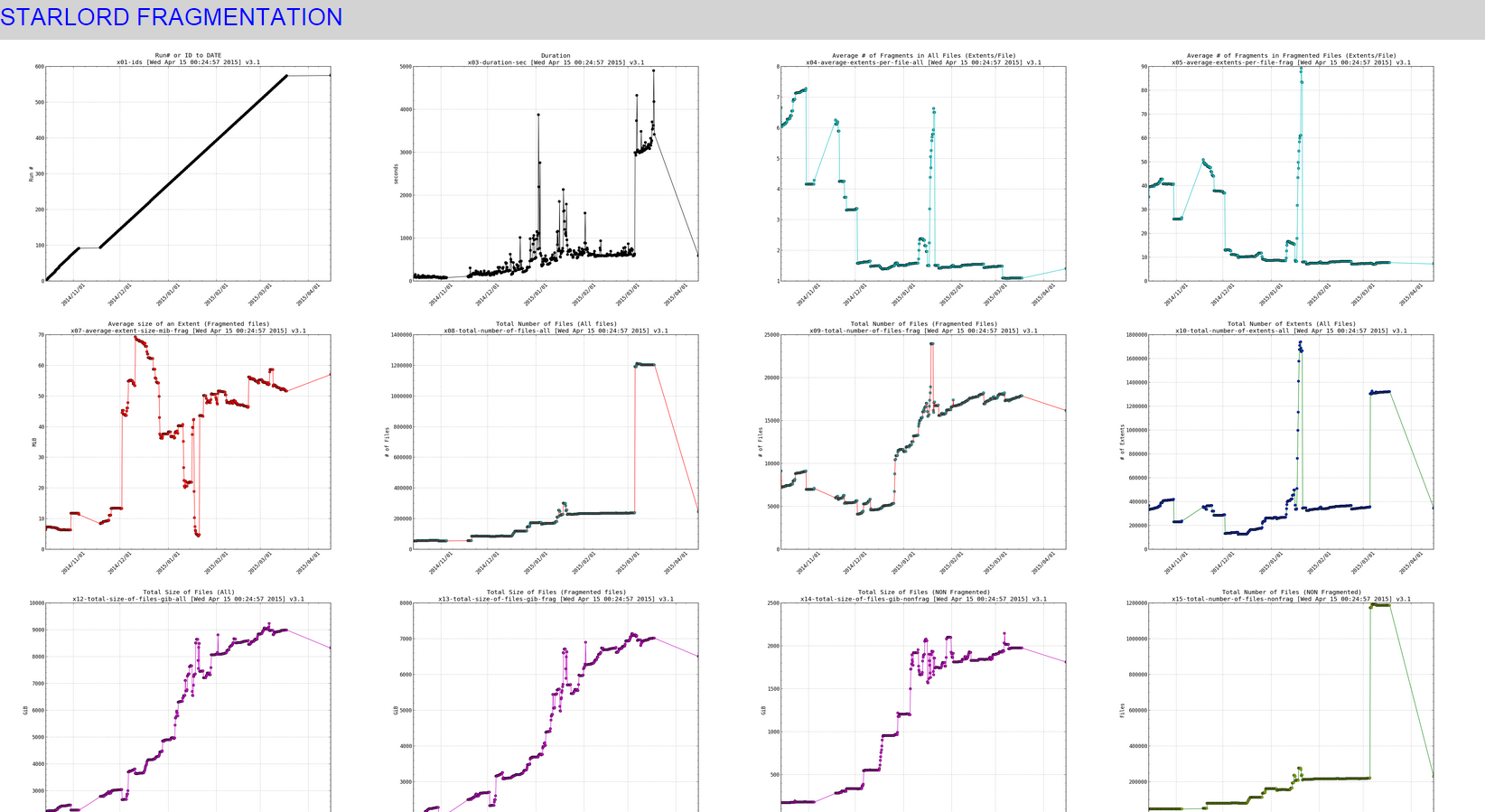When clicking on any of the pics (it would open a really high resolution image that you can zoom in on , to see effect click on the links above to see the live data):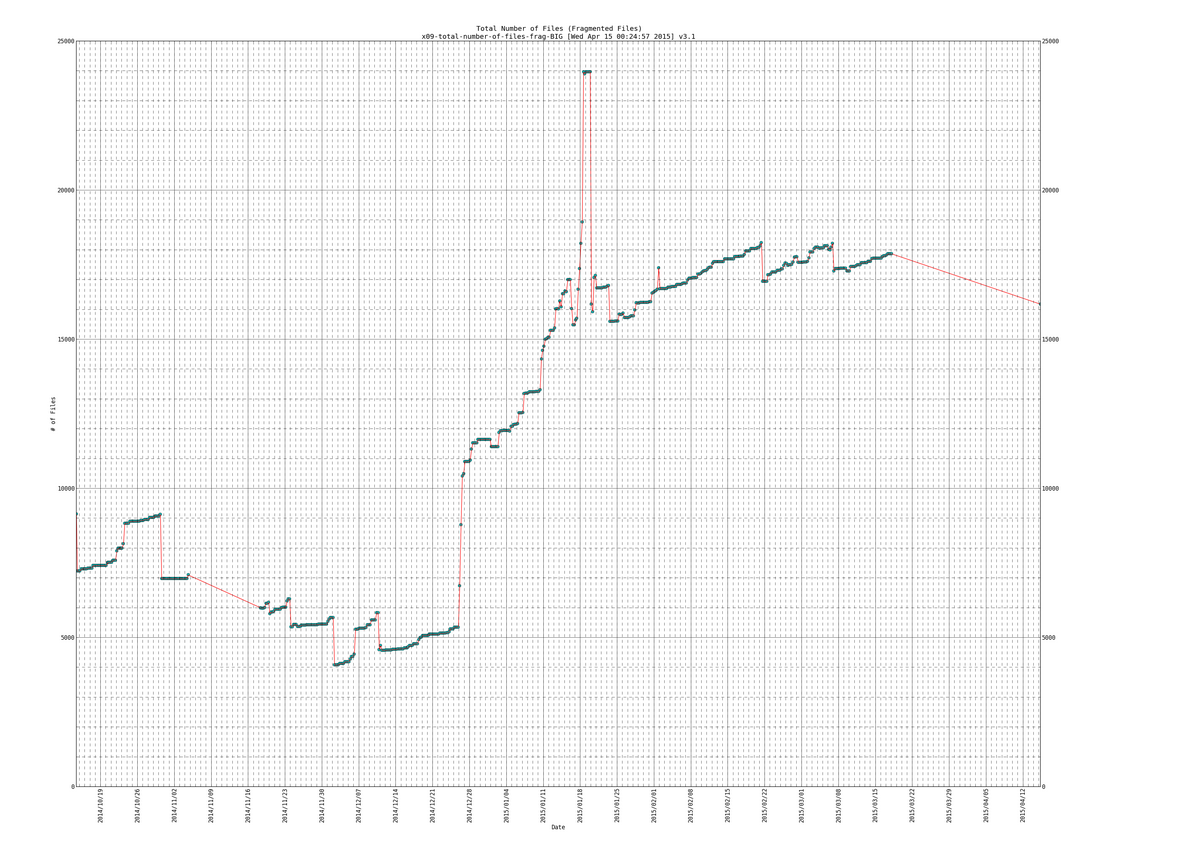SIDENOTE: ignore the part in my plots where the line slopes downward at the end, thats due to the fragmentation scripts data gathering part not working for a while (a month or so) and then picking back up again.

Lines of data that we will be parsing:

Here is the script:

#### EXAMPLE 3 – transfer rates on my iptest server

SIDENOTE: This example has Trend line, so if you want to see that look for the section of code that start with “TRENDLINE” and ends with “TRENDLINE end” (there should be 3 sections like that)

Here is the output (live):

Here is a snapshot of the small plots: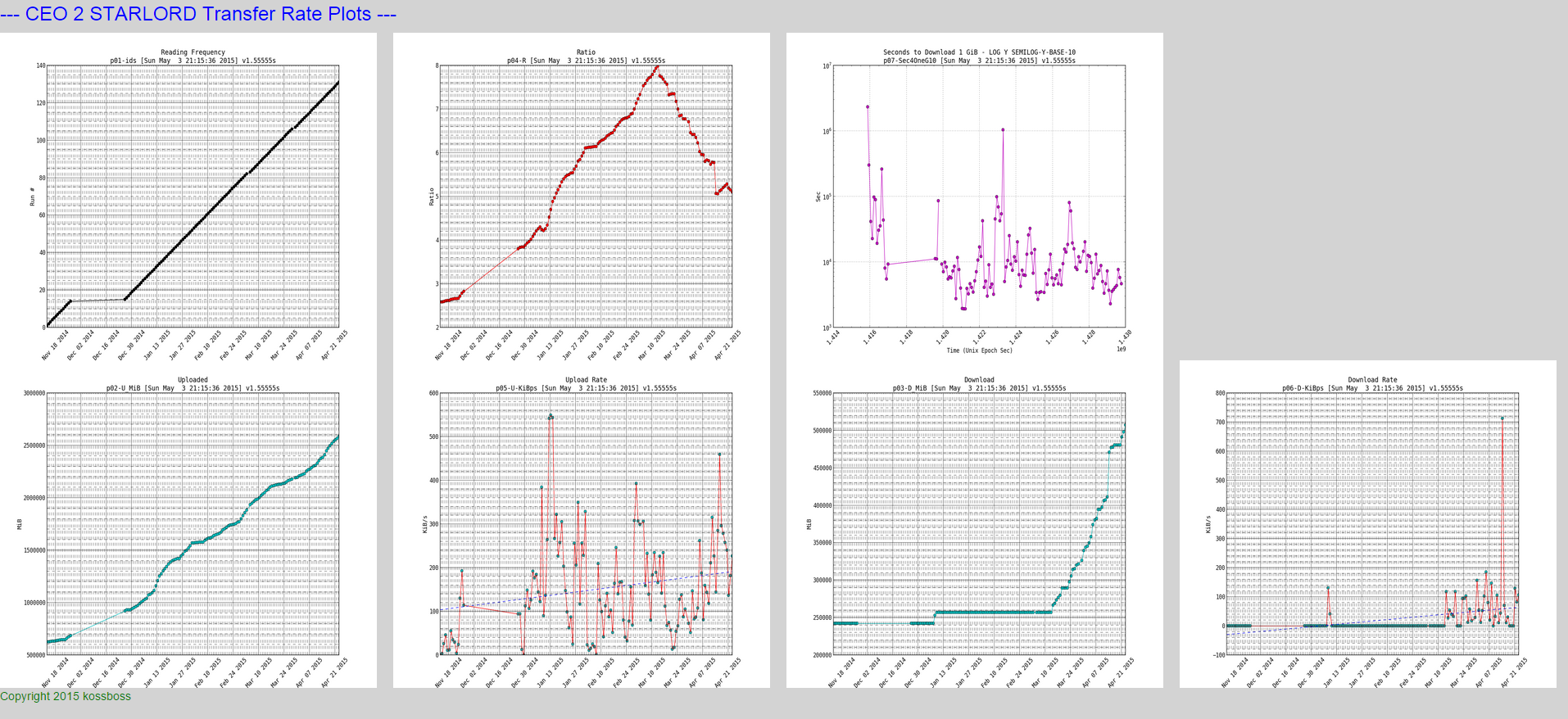NOTE: if you look closely at the download and upload rates you can see a trend line which I will show how to plot in the python script

Here is a snapshot of the big plots: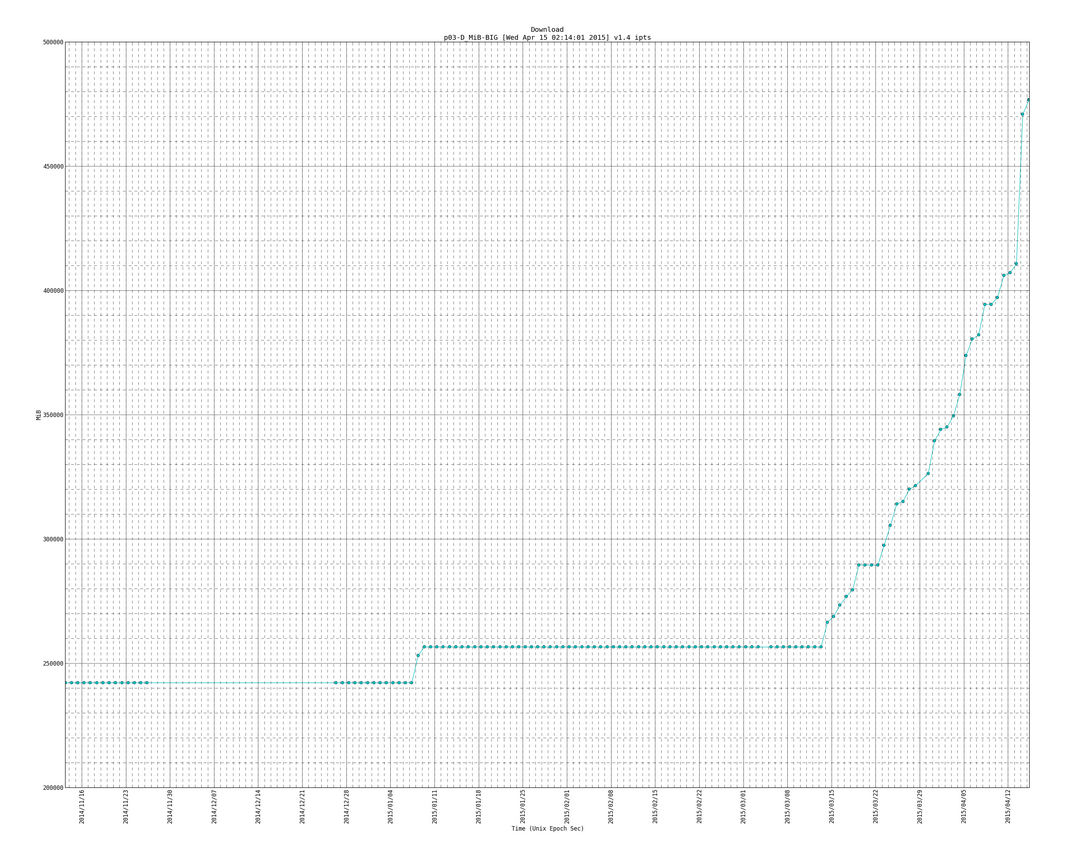Here is an example of the big plot with the trend line (notice the version number is newer than plot above and it has more data entries as this one is newer than the plot directly above):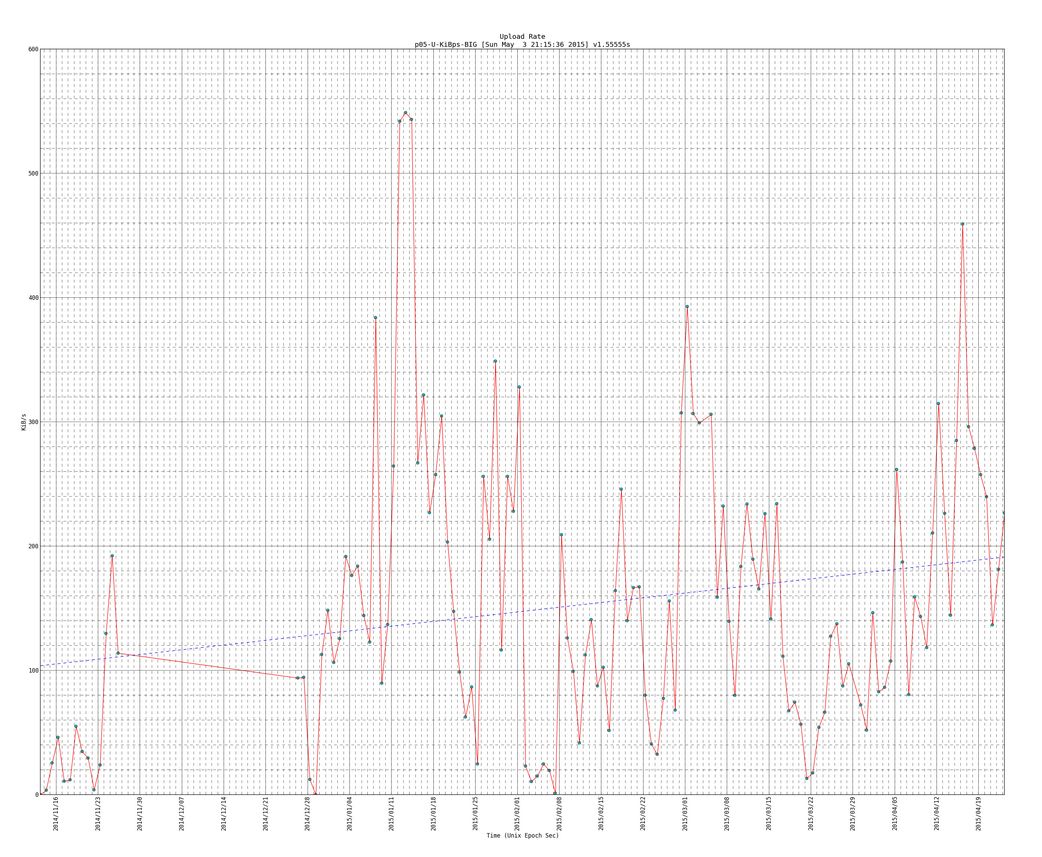SIDENOTE: you will see code for trendlines, which I borrowed from here: http://widu.tumblr.com/post/43624347354/matplotlib-trendline which use the polyfit and poly1d functions (discussed more here: http://www.mathworks.com/help/matlab/ref/polyfit.html and http://docs.scipy.org/doc/numpy/reference/generated/numpy.poly1d.html )

Here is the data that we parsed:

Here is the script:

TO DO:
test example1 and fix any minor mistakes. i wrote it up and it should generally be correct. however there could be minor syntax errors. other than that dont worry example2 & 3 are taken from working scripts that I have been running for months to graph fragmentation and to graph transfers between my servers.